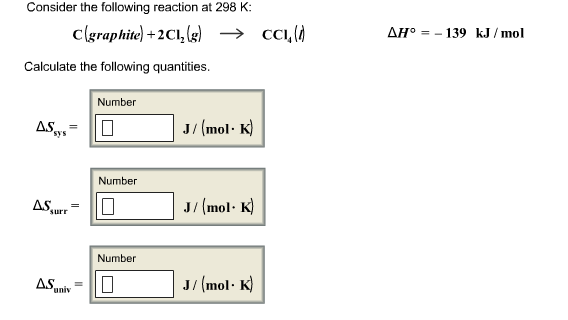# Question & Answer: Consider the following reaction at 298 K: C(graphite) + 2Cl_2(g) rightarrow CCl_4(l) Delta H…..Consider the following reaction at 298 K: C(graphite) + 2Cl_2(g) rightarrow CCl_4(l) Delta H degree = -139 kJ/mol Calculate the following quantities. Delta S_sys = Delta S_surr = Delta S_univ =

a)

Don't use plagiarized sources. Get Your Custom Essay on
Question & Answer: Consider the following reaction at 298 K: C(graphite) + 2Cl_2(g) rightarrow CCl_4(l) Delta H…..
GET AN ESSAY WRITTEN FOR YOU FROM AS LOW AS \$13/PAGE

dSystem = Sproducts – Sreactants

dSystem = s-CCl4 – (S-C + 2*S-Cl2)

dssystem = 214.4 – (5.7 + 2*223.1)

dSsystem = -237.5 J/K-mol

b)

dSsurroundings

Assume:

dSsurroundings = dQsurr/Tsurr

dQsurr = Qsurr

Qsurr = -Qsystem

Qsystem = Hrxn

Qsurr = -(-HRxn) = -(-139) = 139 kJ/mol = 139000 J/mol

dSsurroundings = 139000 J / (298K) = 466.442 J/mol-K

c)

dSsuniverse = dSsystem + dSsurroundings

dSsuniverse = -237.5 + 466.442

dSuniverse = 228.942 J/Kmol